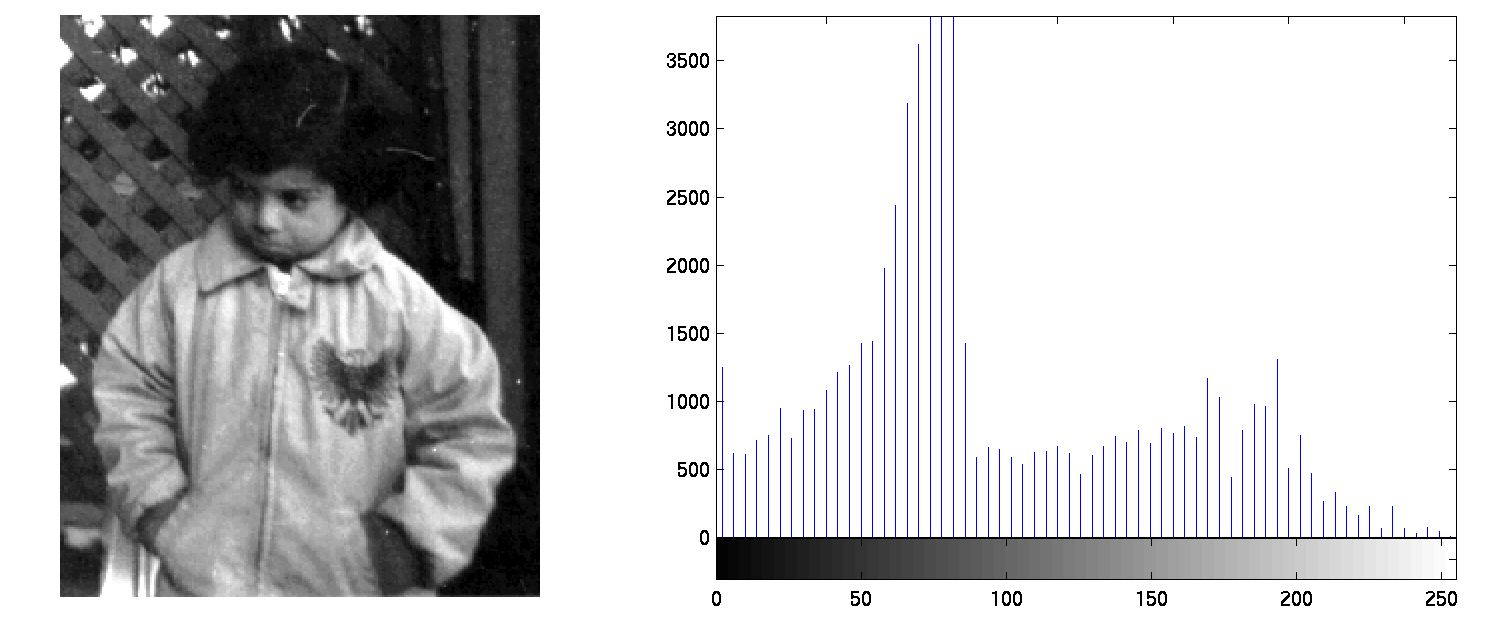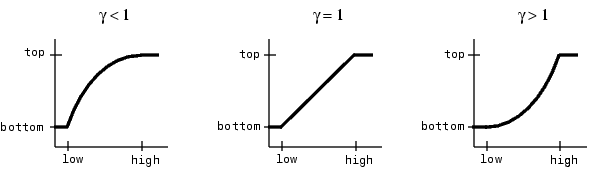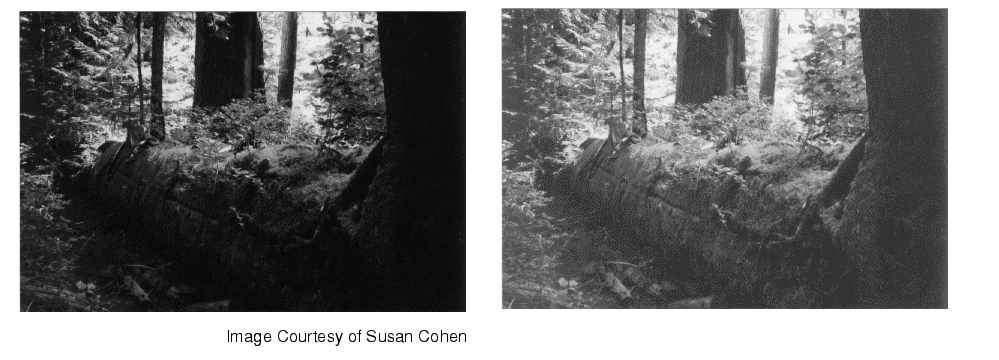Image Processing Toolbox User's GuideAdjusting Intensity Values to a Specified Range

You can adjust the intensity values in an image using the `imadjust` function, where you specify the range of intensity values in the output image.

For example, this code increases the contrast in a low-contrast grayscale image by remapping the data values to fill the entire intensity range [0, 255].

• ```I = imread('pout.tif');
imshow(J)
figure, imhist(J,64)
```

This figure displays the adjusted image and its histogram. Notice the increased contrast in the image, and that the histogram now fills the entire range.You can optionally specify the range of the input values and the output values using `imadjust`. You specify these ranges in two vectors that you pass to `imadjust` as arguments. The first vector specifies the low- and high-intensity values that you want to map. The second vector specifies the scale over which you want to map them.

 Note    Note that you must specify the intensities as values between 0 and 1 regardless of the class of `I`. If `I` is `uint8`, the values you supply are multiplied by 255 to determine the actual values to use; if `I` is `uint16`, the values are multiplied by 65535. To learn about an alternative way to set these limits automatically, see Setting the Adjustment Limits Automatically.

For example, you can decrease the contrast of an image by narrowing the range of the data. In the example below, the man's coat is too dark to reveal any detail. `imadjust` maps the range [0,51] in the `uint8` input image to [128,255] in the output image. This brightens the image considerably, and also widens the dynamic range of the dark portions of the original image, making it much easier to see the details in the coat. Note, however, that because all values above 51 in the original image are mapped to 255 (white) in the adjusted image, the adjusted image appears washed out.

• ```I = imread('cameraman.tif');
imshow(I)
figure, imshow(J)```

Image After Remapping and Widening the Dynamic Range

To use `imadjust`, you must typically perform two steps:

1. View the histogram of the image to determine the intensity value limits.
2. Specify these limits as a fraction between 0.0 and 1.0 so that you can pass them to `imadjust` in the `[low_in high_in]` vector.

For a more convenient way to specify these limits, use the `stretchlim` function. (The `imadjust` function uses `stretchlim` for its simplest syntax, `imadjust(I)`.)

This function calculates the histogram of the image and determines the adjustment limits automatically. The `stretchlim` function returns these values as fractions in a vector that you can pass as the `[low_in high_in]` argument to `imadjust`; for example:

• ```I = imread('rice.png');
```

By default, `stretchlim` uses the intensity values that represent the bottom 1% (0.01) and the top 1% (0.99) of the range as the adjustment limits. By trimming the extremes at both ends of the intensity range, `stretchlim` makes more room in the adjusted dynamic range for the remaining intensities. But you can specify other range limits as an argument to `stretchlim`. See the `stretchlim` reference page for more information.

Gamma Correction

`imadjust` maps `low` to `bottom`, and `high` to `top`. By default, the values between `low` and `high` are mapped linearly to values between `bottom` and `top`. For example, the value halfway between `low` and `high` corresponds to the value halfway between `bottom` and `top`.

`imadjust` can accept an additional argument that specifies the gamma correction factor. Depending on the value of gamma, the mapping between values in the input and output images might be nonlinear. For example, the value halfway between `low` and `high` might map to a value either greater than or less than the value halfway between `bottom` and `top`.

Gamma can be any value between 0 and infinity. If gamma is 1 (the default), the mapping is linear. If gamma is less than 1, the mapping is weighted toward higher (brighter) output values. If gamma is greater than 1, the mapping is weighted toward lower (darker) output values.

The figure below illustrates this relationship. The three transformation curves show how values are mapped when gamma is less than, equal to, and greater than 1. (In each graph, the x-axis represents the intensity values in the input image, and the y-axis represents the intensity values in the output image.)Plots Showing Three Different Gamma Correction Settings

The example below illustrates gamma correction. Notice that in the call to `imadjust`, the data ranges of the input and output images are specified as empty matrices. When you specify an empty matrix, `imadjust` uses the default range of [0,1]. In the example, both ranges are left empty; this means that gamma correction is applied without any other adjustment of the data.

• ```[X,map] = imread('forest.tif')
I = ind2gray(X,map);Intensity Adjustment Histogram Equalization